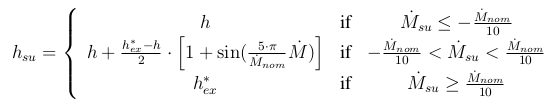# Discretization Schemes

A large part of the models proposed in ThermoCycle are based on the finite volume discretization (as opposed to e.g. moving-boundaries models). The flow is discretized into N cells in which the energy and mass conservation equations are applied. The momentum can generally be neglected.

Two types of variables can be distinguished: cell variables and node variables. Node variables are distinguished by the ”su” (supply) and ”ex” (exhaust) subscripts, and correspond to the inlet and outlet nodes of each cell. The ”*” exponent indicates a value relative to the adjacent cell.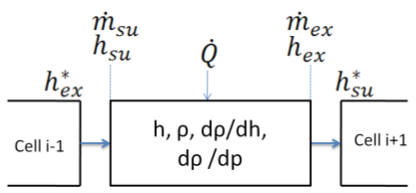The mass balance is expressed as a function of the two differentiated state variable (p and h):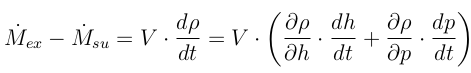The energy balance is expressed by: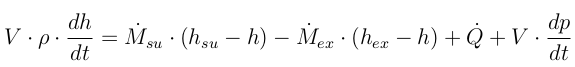The relation between the cell and node values depends on the discretization scheme. In ThermoCycle two schemes are implemented, the central difference scheme and the upwind scheme. Since the model accounts for flow reversal, a conditional statement is added depending on the flow rates at the inlet and outlet nodes.

For the central differences scheme: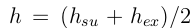The supply enthalpy can therefore be computed by (the equivalent equation applies to the exhaust enthalpy):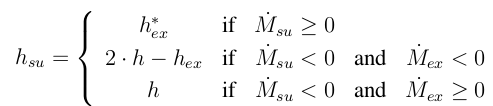In the upwind scheme the equation becomes: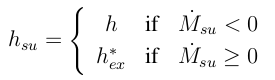The overall flow model is finally obtained by interconnecting several cells in series. This kind of discretization corresponds to a staggered grid, i.e. the thermodynamics states and the state variables (the enthalpies) are calculated inside the cells and the node values (”su” and ”ex”) are deduced. In a collocated grid, on the contrary, the state variables are the node variables and the cell variables are deduced.

# In ThermoCycle

The discretization schemes presented above are implemented in the Cell1Dim and Flow1Dim components of ThermoCycle.

The available options in the dropdown menu are the following:

• Central Difference Scheme – Basic. This implementation of the central difference scheme can only handle unidirectional flow. This scheme is more accurate than the upwind scheme due to the averaging of the node values: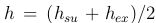• Central Differences Scheme – Allows Reverse Flow. In this expression, an if statement is added to compute the proper node value as a function of the flow rate direction. It should be noted that reverse flows are possible with this method, but flow reversals generate numeric errors.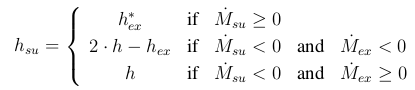• Upwind Scheme – Basic. Implementation of the upwind scheme for unidirectional flow: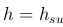• Upwind Scheme – Allows Flow reversal. An if statement is added to handle reverse flows. Flow reversals and zero flows can also be handled with this method, provided that proper numeric methods are selected to avoid chattering or stiff systems.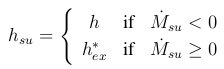• Upwind Scheme with Smoothing. This scheme is variation of the previous one aimed at avoiding the discontinuity in the node enthalpy when a flow reversal occurs. A smooth transition function is used to switch from one value of the enthalpy to another. It should be noted that this method generates a highly nonlinear system of equations that can be difficult to handle for the solver.